## Thursday, 7 August 2014

### chapter-11 -worked-out-examples-4

 Example: 7
 A pair of dice is rolled until a sum of either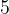$5$ or$7$ is obtained. Find the probability that$5$ comes before$7$.
 Solution: 7
A sum of$5$ can be obtained in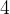$4$ ways, namely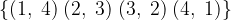$\{ (1,\;4)\;(2,\;3)\;(3,\;2)\;(4,\;1)\}$
from a total number of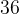$36$ ways of throwing a pair of dice. If we let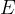$E$ denote the event$5$ of obtaining a sum of$5$, we have$P(E) = \dfrac{4}{{36}} = \dfrac{1}{9}$
Similarly, let the event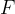$F$ be that of obtaining a sum of$7$; this can happen in$6$ ways, namely$\left\{ {(1,\;6)\;(2,\;5)\;(3,\;4)\;(4,\;3)\;(5,\;2)\;(6,\;1)} \right\}$
so that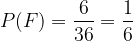$P(F) = \dfrac{6}{{36}} = \dfrac{1}{6}$
Finally, if we let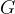$G$ be the event of obtaining neither a$5$ or a$7$, we have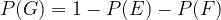$P(G) = 1 - P(E) - P(F)$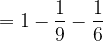$= 1 - \dfrac{1}{9} - \dfrac{1}{6}$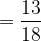$= \dfrac{{13}}{{18}}$
Now, we want a sum of$5$ to come before a sum of$7$. Think about how this can happen. Every time you roll the pair of dice, you should either get a sum of$5$ or you should get neither a sum of$5$ nor$7$.
Therefore, the following (mutually exclusive) sequences of throws leads us to a sum of$5$before a sum of$7$.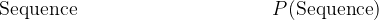${\rm{Sequence}} \,\,\,\,\,\,\,\,\,\,\,\,\,\,\,\,\,\,\,\,\,\, \,\,\,\,\, \,\,\,\,\,\,\,\,\,\,\,\,\,\,\,\,\,\,\,\,\,\,\,\,\,\,\,\,\,\,\,\,P({\rm{Sequence}})$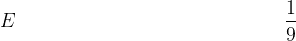$E \,\,\,\,\,\,\,\,\,\,\,\,\,\,\,\,\,\,\,\,\,\,\,\,\,\,\,\,\,\,\,\,\,\,\,\,\,\,\,\,\,\,\,\,\,\,\,\,\,\,\,\,\,\,\,\,\,\,\,\,\,\,\,\,\,\,\,\,\,\,\,\,\,\,\,\,\,\,\,\,\,\dfrac{1}{9}$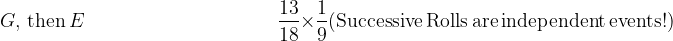$G, \,{\rm{then}}\,E\,\,\,\,\,\,\,\,\,\,\,\,\,\,\,\,\,\,\,\,\,\,\,\,\,\,\,\,\,\,\,\,\,\,\,\,\,\,\,\,\,\,\,\,\,\,\,\,\,\,\,\,\,\,\,\,\,\, \dfrac{{13}}{{18}} \times \dfrac{1}{9}({\rm{Successive \,Rolls\, are\, independent\, events!}})$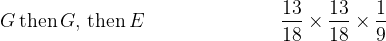$G \,{\rm{then}} \,G,\, {\rm{then}} \, E \,\,\,\,\,\,\,\,\,\,\,\,\,\,\,\,\,\,\,\,\,\,\,\,\,\,\,\,\,\,\,\,\,\,\,\,\,\,\,\,\,\dfrac{{13}}{{18}} \times \dfrac{{13}}{{18}} \times \dfrac{1}{9}$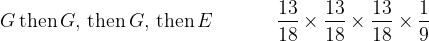$G\,{\rm{then}}\, G,\, {\rm{then}}\, G, \,{\rm{then}} \, E \,\,\,\,\,\,\,\,\,\,\,\,\,\,\,\,\,\,\, \dfrac{{13}}{{18}} \times \dfrac{{13}}{{18}} \times \dfrac{{13}}{{18}} \times \dfrac{1}{9}$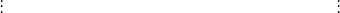$\,\,\,\,\,\,\,\,\,\,\,\,\,\,\,\,\,\,\,\vdots \,\,\,\,\,\,\,\,\,\,\,\,\,\,\,\,\,\,\,\,\,\,\,\,\,\,\,\,\,\,\,\,\,\,\,\,\,\,\,\,\,\,\,\,\,\,\,\,\,\,\,\,\,\,\,\,\,\,\,\,\,\, \,\,\,\,\,\,\,\,\,\,\,\,\,\,\,\,\,\,\,\,\,\,\,\,\,\,\,\,\,\,\,\,\,\,\,\,\,\,\vdots$
The required probability is obtained by adding the terms in the right column.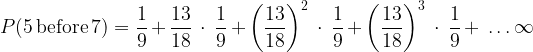$P(5\,{\rm{before}}\,7)=\dfrac{1}{9} + \dfrac{{13}}{{18}}\; \cdot \;\dfrac{1}{9} + {\left( {\dfrac{{13}}{{18}}} \right)^2}\; \cdot \;\dfrac{1}{9} + {\left( {\dfrac{{13}}{{18}}} \right)^3}\; \cdot \;\dfrac{1}{9} + \;\ldots \infty$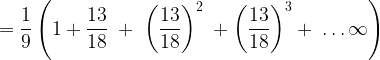$= \dfrac{1}{9}\left( {1 + \dfrac{{13}}{{18}}\; + \;{{\left( {\dfrac{{13}}{{18}}} \right)}^2}\; + {{\left( {\dfrac{{13}}{{18}}} \right)}^3} + \;\ldots \infty } \right)$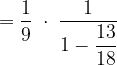$= \dfrac{1}{9}\; \cdot \;\dfrac{1}{{1 - \dfrac{{13}}{{18}}}}$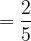$= \dfrac{2}{5}$
In passing, note that the probability of obtaining a$7$ before$5$ is simply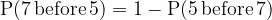${\rm{P (7 \,{\rm{before}}\, 5) = 1 - P(5\,{\rm{ before}}\, 7)}}$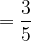$= \dfrac{3}{5}$
Can you appreciate why obtaining a$7$ before$5$ is more likely than obtaining a$5$ before$7$?
 Example: 8
 Two unit squares are chosen at random from a standard chessboard. What is the probability that the two squares have exactly one corner in common?
 Solution: 8
 The total number of ways of selecting two squares from a standard chessboard is simply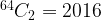${}^{64}{C_2} = 2016$. Now, let us find the number of ways of selecting a pair with exactly one corner in common. For that, consider the following figure.It is immediately evident that the number of favorable ways is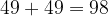$49 + 49 = 98$. The required probability is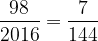$\dfrac{{98}}{{2016}} = \dfrac{7}{{144}}$